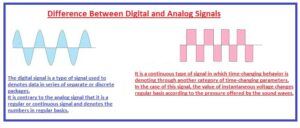Hi, readers welcome to the new post. In this post, we will have a detailed look at Difference Between Digital and Analog Signals. Both of these are types of signals that used to transmit data and information from one point to another. In analog signal, data is transferred continuously which varies according to the periods while in digital data is a move-in separate form. If we do a comparison between these two we will come to know that digital signal exists in square shape while analog in sine waves.

In this post, we will cover their basic with details so let get started.

## Difference Between Digital and Analog Signals

Analog Signal

• It is a continuous type of signal in which time-changing behavior is denoting through another category of time-changing parameters.
• In the case of this signal, the value of instantaneous voltage changes regular basis according to the pressure offered by the sound waves.
• Analog signal generally denoted for the electrical signal with that other networks like mechanical, hydraulic also part of analog signal
• In this signal there use of the medium to transmit the signal in form of data. An example of it is an aneroid barometer.
• In the electrical signal, the voltage-current of the signal can be changed to denotes the data.
• In this category of signal, there is the usage of any feature of medium to transmit the info of signal.
• Such as in the case of a signal that is analog signal is transmitted through voltage current and frequency if any variation occurs in it.
• Data can be transmitted through this signaling there is changes that occur in the physical variable like temperature, pressure, etc.
• There is the conversion of physical parameters in the analog signal through the use of a device called a transducer.
• In this signal, there is the occurrence of distortion due to the signal process and some other related parameters that cause the SNR.
• This signal-to-noise ratio caused the signal to degrade when it transmitted.Digital Signal

• The digital signal is a type of signal used to denotes data in series of separate or discrete packages.
• It is contrary to the analog signal that it is a regular or continuous signal and denotes the numbers in regular basics.
• In digital signal denotes the data in separate groups of the analog signal. Every group or band consists of similar data.
• In mostly digital circuitry the signal consists of 2 certain parameters which is known as binary or logics.
• Those are denoted through 2 voltage groups first is zero and the second one is high or close to supply.
• Normally denotes s boolean domina. It also denotes false and true high or low according to conditions.
• There are 2 main conditions of digital signals first one called multivalued logic and the second one is three values logic.
• In this signal, the physical parameters denoting the data can be variable values like current or in some cases voltage.
• These signals are normally employed in digital electronics devices computer and for sending od data from one place to another.
• In the case of digital electronics digital signals called pulse trains and generated through the use of MOSFET

That is a detailed post about Difference Between Digital and Analog Signals. If you have any query ask in the comments. Thanks for reading have a nice day.